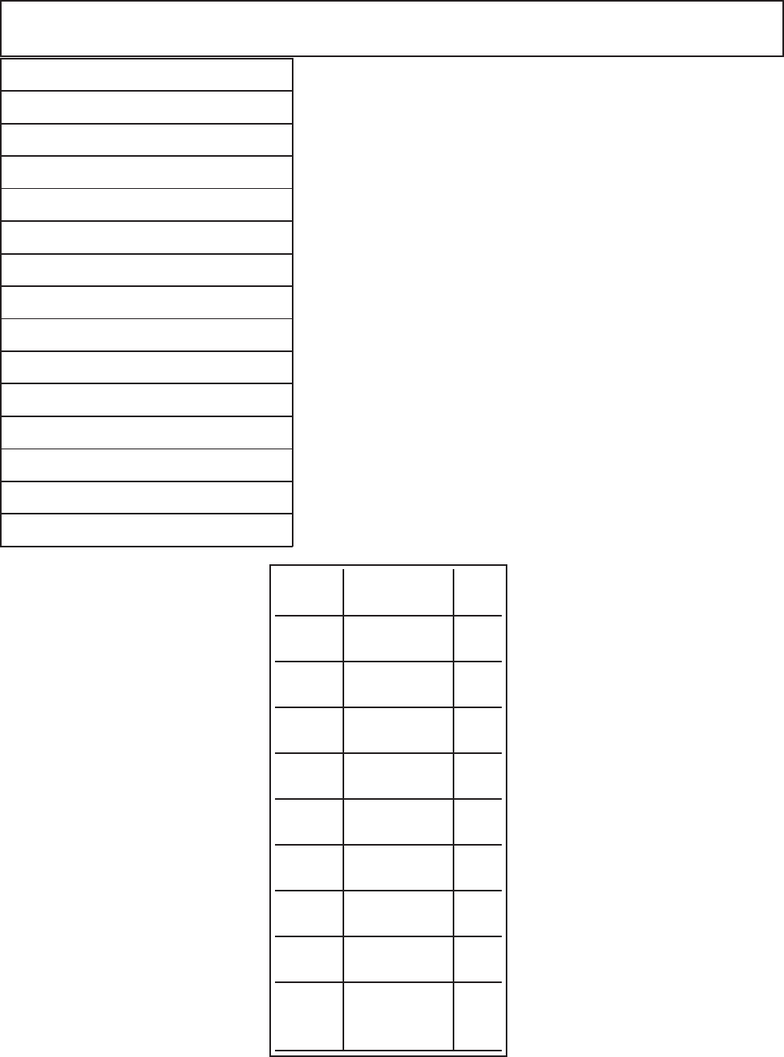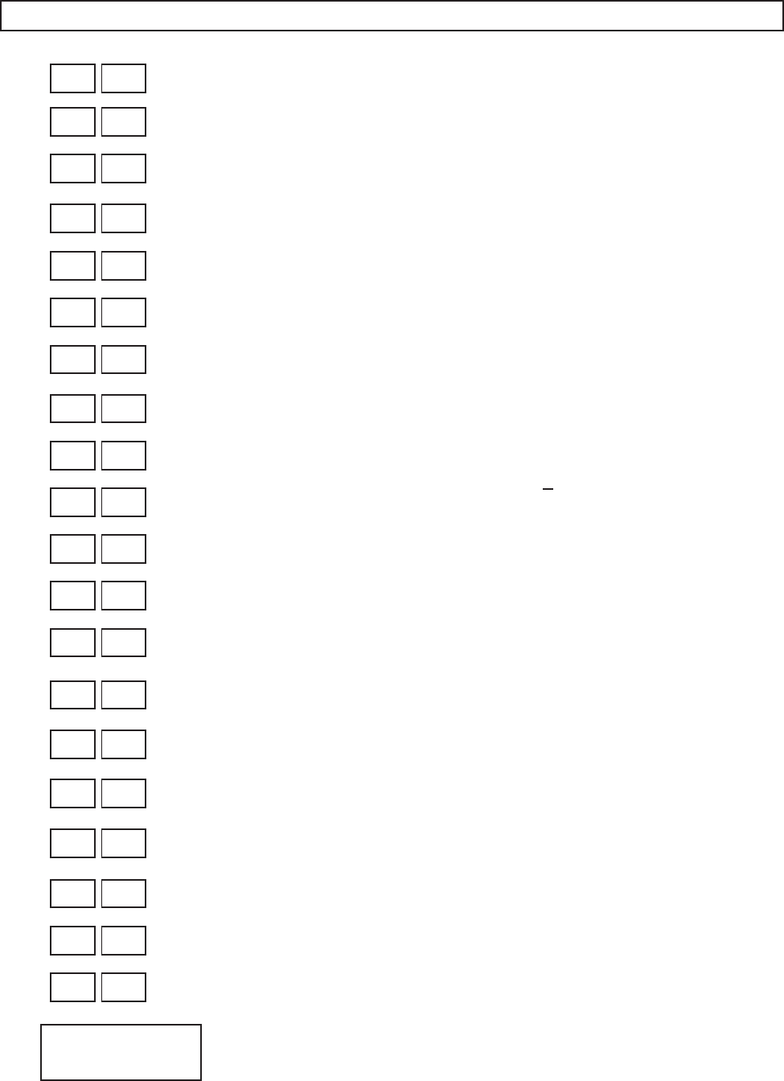Study Guides (400,000)
US (230,000)
Harvard (700)
MATH (400)
MATH 21A (100)
All (100)
Midterm

# MATH 21A Harvard 21a Fall 14Midterm 1Exam

Department
Mathematics
Course Code
MATH 21A
Professor
All
Study Guide
Midterm

This preview shows pages 1-2. to view the full 7 pages of the document.9/30/2014 FIRST HOURLY Math 21a, Fall 2014
Name:
MWF 9 Oliver Knill
MWF 9 Chao Li
MWF 10 Gijs Heuts
MWF 10 Yu-Wen Hsu
MWF 10 Yong-Suk Moon
MWF 11 Rosalie Belanger-Rioux
MWF 11 Gijs Heuts
MWF 11 Siu-Cheong Lau
MWF 12 Erick Knight
MWF 12 Kate Penner
TTH 10 Peter Smillie
TTH 10 Jeﬀ Kuan
TTH 10 Yi Xie
TTH 11:30 Jeﬀ Kuan
TTH 11:30 Jameel Al-Aidroos
Start by printing your name in the above box
the left.
Do not detach pages from this exam packet
or unstaple the packet.
ible for the grader cannot be given credit.
Show your work. Except for problems 1-3
or problem 9, we need to see details of your
computation.
All functions can be diﬀerentiated arbitrarily
often unless otherwise speciﬁed.
No notes, books, calculators, computers, or
other electronic aids can be allowed.
You have 90 minutes to complete your work.
1 20
2 10
3 10
4 10
5 10
6 10
7 10
8 10
9 10
Total: 100
1

Only pages 1-2 are available for preview. Some parts have been intentionally blurred.Problem 1) (20 points) No justiﬁcations are needed.
1) T F The surface x2+y2+z2=1 is a one-sheeted hyperboloid.
2) T F The equation y= 3x+ 2 in space deﬁnes a plane.
3) T F Whenever |~r (t)|= 1 then |~
T(t)|= 1.
4) T F The length of the vector projection Proj~v(~w) is smaller than or equal to the
length of ~w.
5) T F The velocity vector of ~r(t) = ht, t, tiat time t= 2 is the same as the velocity
vector at t= 1.
6) T F If ~v ×~w =~w ×~v then ~v, ~w are parallel.
7) T F The vector h−2,1,0iis perpendicular to the line h1 + t, 2t, 3ti.
8) T F The point given in spherical coordinates as ρ= 3, φ = 0, θ =πis the same
point as the point ρ= 3, φ = 0, θ = 0.
9) T F The parametrized curve ~r(t) = h0,3 cos(t),5 sin(t)iis an ellipse.
10) T F The curvature of the line ~r(t) = ht, t, tiis 3 everywhere.
11) T F If |~v ×~w|=~v ·~w then either ~v is parallel to ~w or perpendicular to ~w.
12) T F If the dot product between two unit vectors ~v, ~w is 1, then ~v =~w.
13) T F Writing ~
k=h0,0,1i, we have |(~
k×~v)×~w| ≤ |~v||~w|for all vectors ~v, ~w.
14) T F The curvature of a curve ~r(t) is given by κ(t) = |~
T(t)|/|~r (t)|. If |~
r(t)|= 1
for all times, then κ(t) = |~r ′′(t)|.
15) T F The arc length of the curve hsin(t/2),0,cos(t/2)ifrom t= 0 to t= 2πis
equal to 2π.
16) T F If L, K are skew lines in space, there is a unique plane which is equidistant
from L, K.
17) T F The curve ~r(t) = ht, t2,1tiis the intersection curve of a plane x+z= 1
and y=x2
18) T F The lines ~r1(t) = h5 + t, 3t, 2tiand ~r2(t) = h6t, 2 + t, 12tiintersect
at (6,2,1) perpendicularly.
19) T F The vector h3/13,12/13,4/13iis a unit vector.
20) T F ~v ×(~v ×~u) = ~
0 for all vectors ~u, ~v.
Total
2